# Chemical Kinetics

8 de Jan de 2011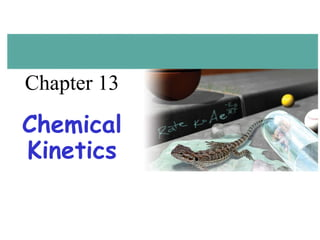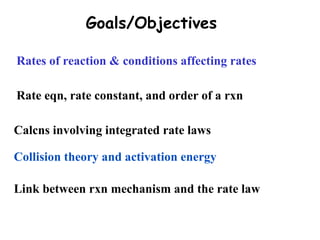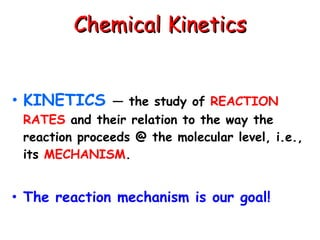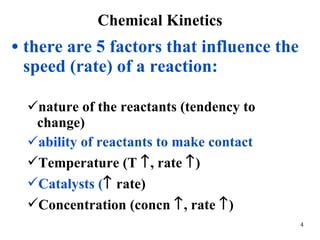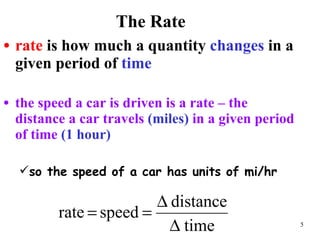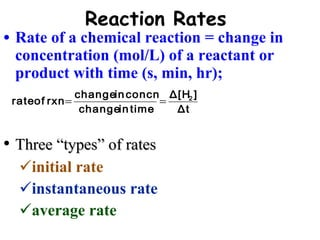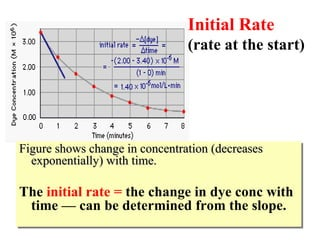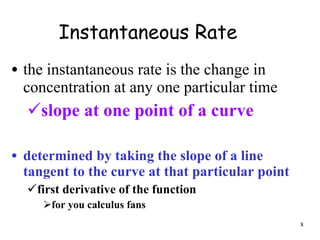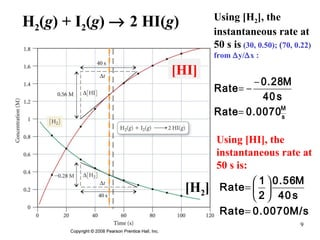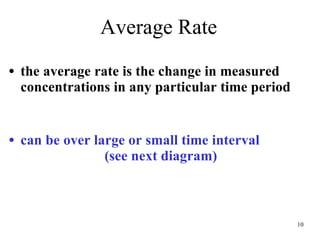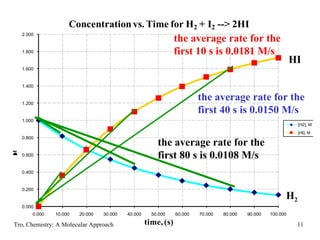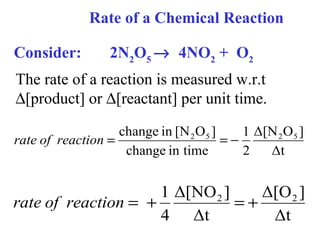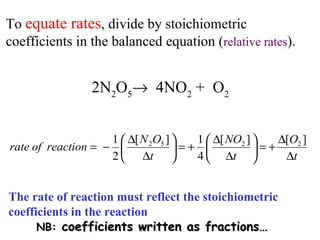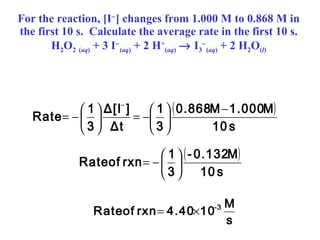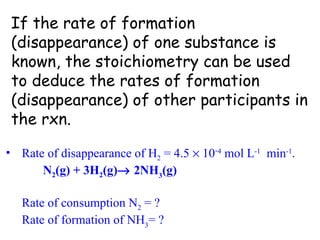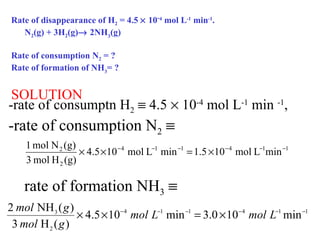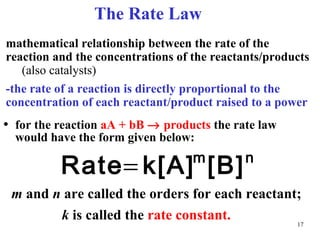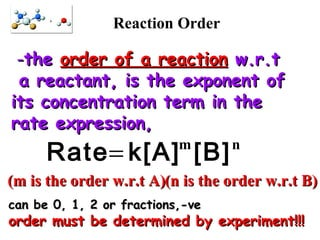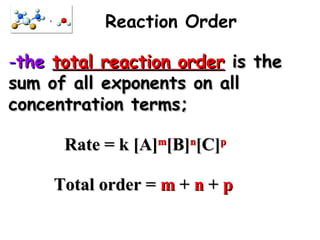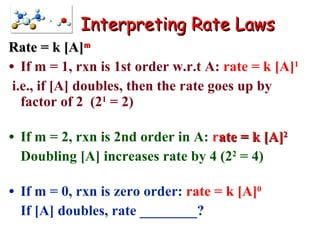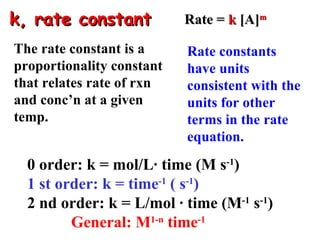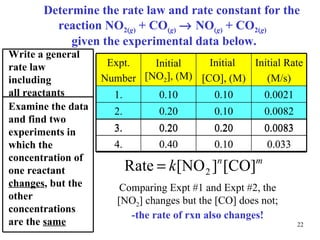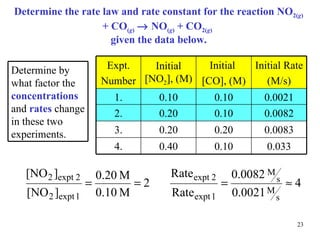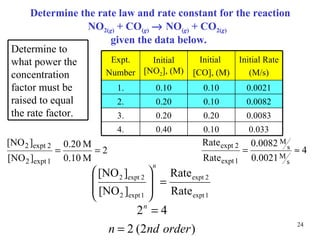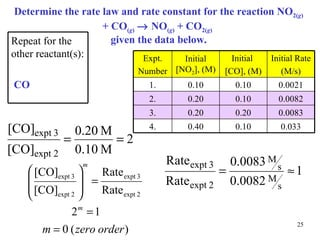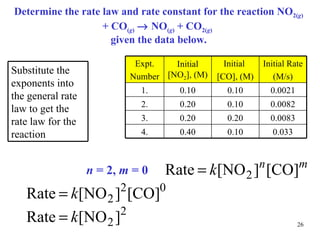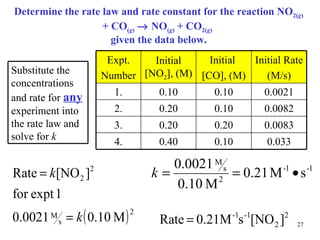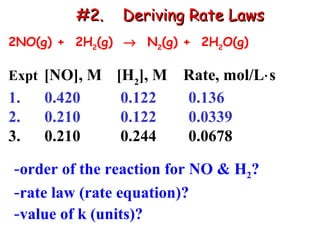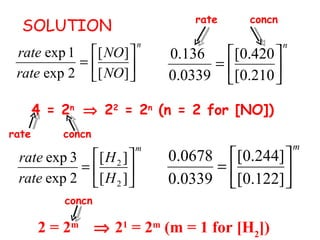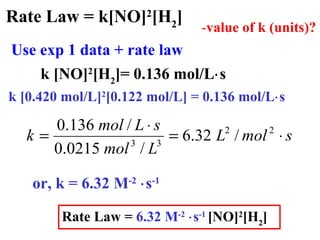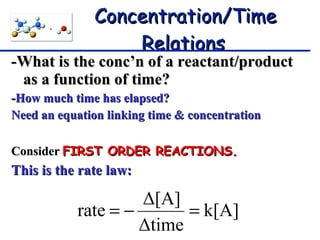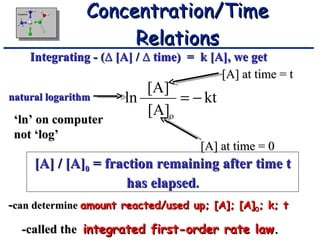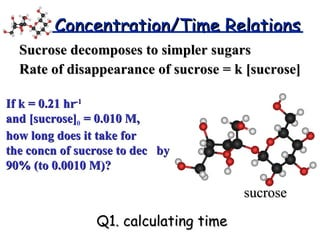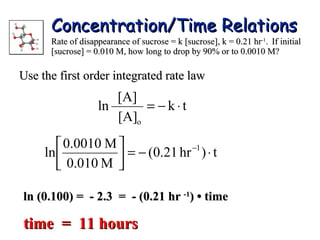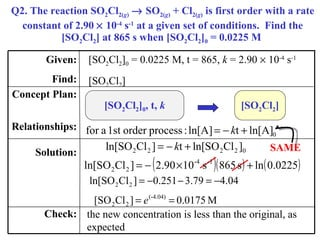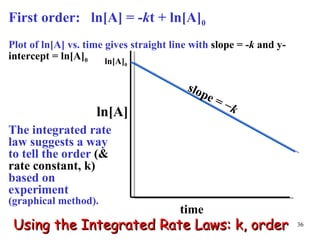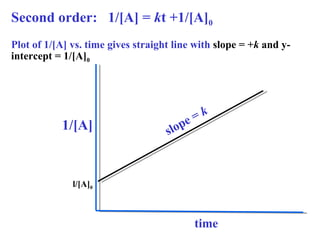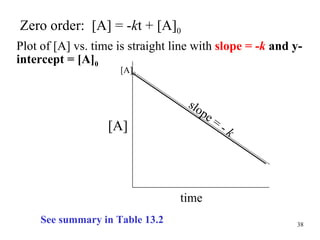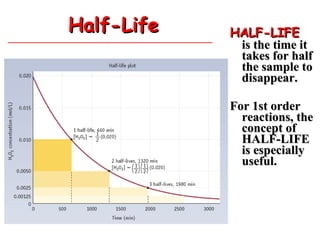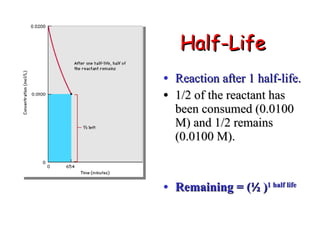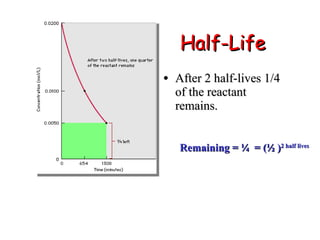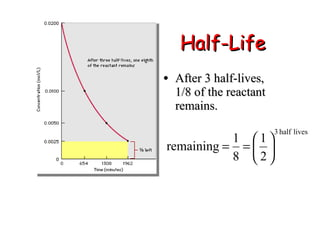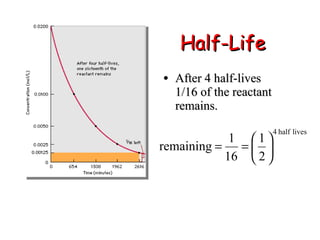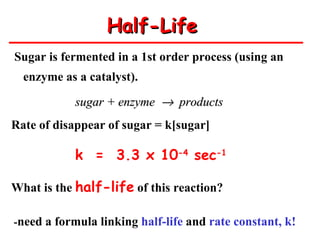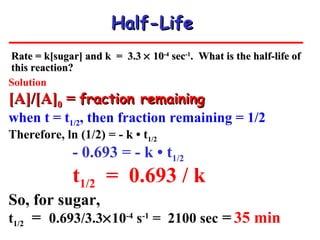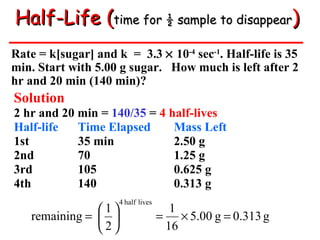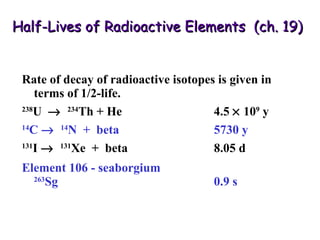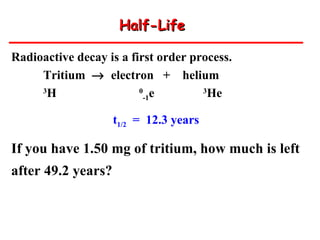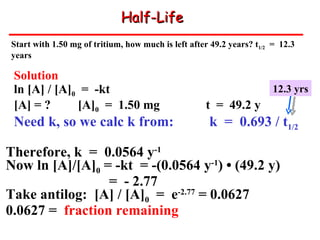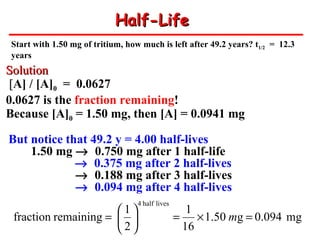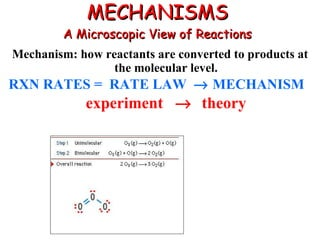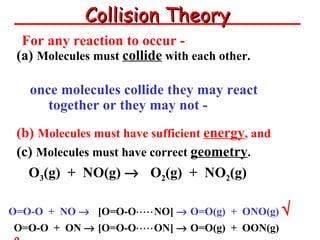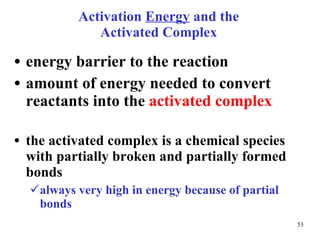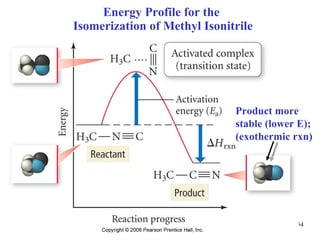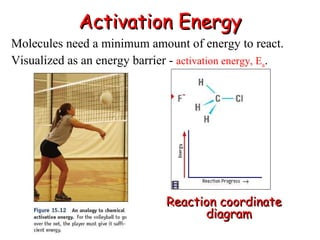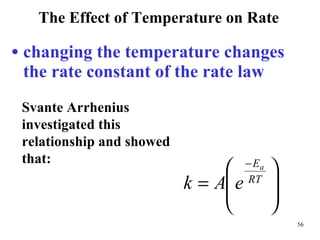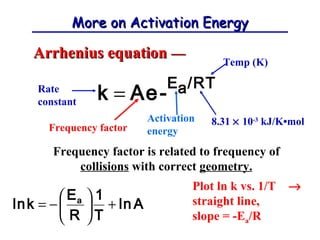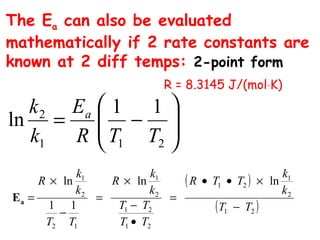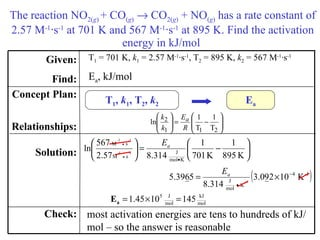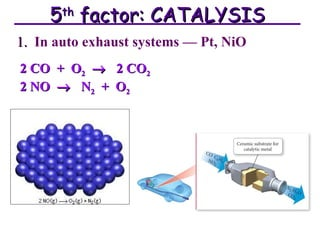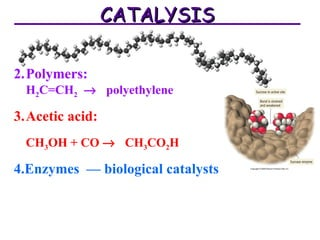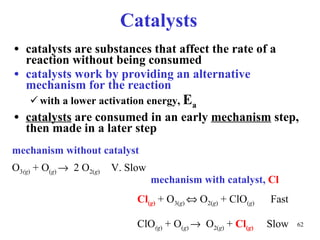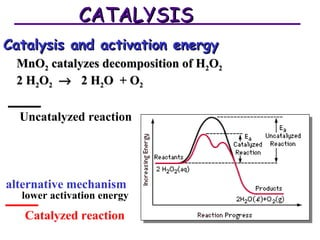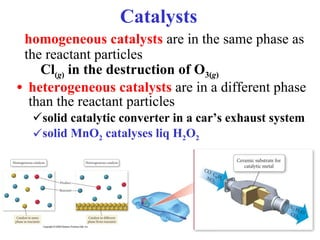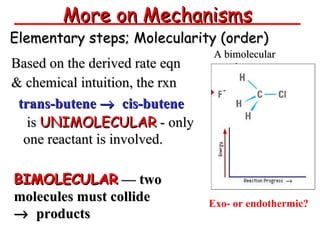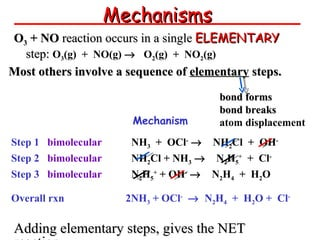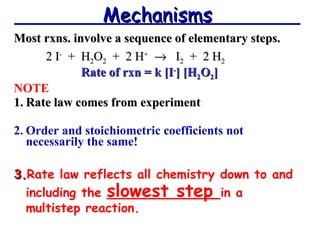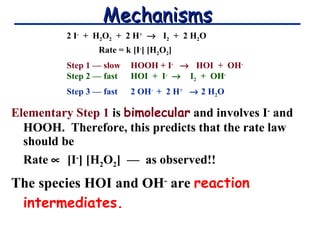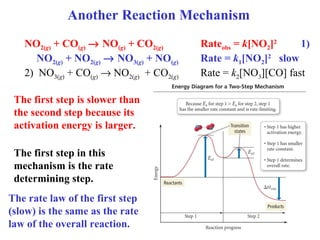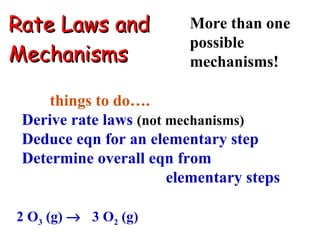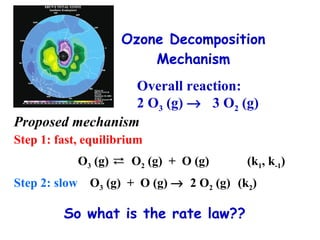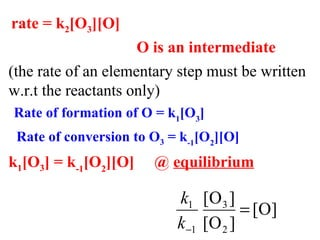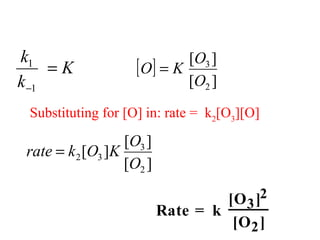1 de 74

### Chemical Kinetics

• 2. Goals/Objectives Rates of reaction & conditions affecting rates Rate eqn, rate constant, and order of a rxn Calcns involving integrated rate laws Collision theory and activation energy Link between rxn mechanism and the rate law
• 3.
• 4.
• 5.
• 6.
• 7. Figure shows change in concentration (decreases exponentially) with time. The initial rate = the change in dye conc with time — can be determined from the slope. Initial Rate (rate at the start)
• 8.
• 9. H 2 ( g ) + I 2 ( g )  2 HI( g ) Using [H 2 ], the instantaneous rate at 50 s is (30, 0.50); ( 70, 0.22) from  y/  x : Using [HI], the instantaneous rate at 50 s is: [H 2 ] [HI]
• 10.
• 11. Tro, Chemistry: A Molecular Approach the average rate for the first 10 s is 0.0181 M/s the average rate for the first 40 s is 0.0150 M/s the average rate for the first 80 s is 0.0108 M/s HI H 2
• 12. Consider: 2N 2 O 5  4NO 2 + O 2 The rate of a reaction is measured w.r.t  [product] or  [reactant] per unit time. Rate of a Chemical Reaction
• 13. To equate rates , divide by stoichiometric coefficients in the balanced equation ( relative rates ). 2N 2 O 5  4NO 2 + O 2 The rate of reaction must reflect the stoichiometric coefficients in the reaction NB: coefficients written as fractions…
• 14. For the reaction, [I  ] changes from 1.000 M to 0.868 M in the first 10 s. Calculate the average rate in the first 10 s. H 2 O 2 ( aq ) + 3 I  ( aq ) + 2 H + ( aq )  I 3  ( aq ) + 2 H 2 O ( l )
• 15.
• 16. Rate of disappearance of H 2 = 4 .5  10 - 4 mol L -1 min -1 . N 2 (g) + 3H 2 (g)  2NH 3 (g)   Rate of consumption N 2 = ? Rate of formation of NH 3 = ? SOLUTION -rate of consumptn H 2  4.5  10 -4 mol L -1 min -1 , -rate of consumption N 2  rate of formation NH 3 
• 17.
• 18. - the order of a reaction w.r.t a reactant, is the exponent of its concentration term in the rate expression, (n is the order w.r.t B) Reaction Order (m is the order w.r.t A) can be 0, 1, 2 or fractions,-ve order must be determined by experiment!!!
• 19. Rate = k [A] m [B] n [C] p Total order = m + n + p - the total reaction order is the sum of all exponents on all concentration terms; Reaction Order
• 20.
• 21. k, rate constant The rate constant is a proportionality constant that relates rate of rxn and conc’n at a given temp. Rate constants have units consistent with the units for other terms in the rate equation. Rate = k [A] m 0 order: k = mol/L· time (M s -1 ) 1 st order: k = time -1 ( s -1 ) 2 nd order: k = L/mol · time (M -1 s -1 ) General: M 1-n time -1
• 22. Determine the rate law and rate constant for the reaction NO 2( g ) + CO ( g )  NO ( g ) + CO 2( g ) given the experimental data below. Comparing Expt #1 and Expt #2, the [NO 2 ] changes but the [CO] does not; -the rate of rxn also changes! Write a general rate law including all reactants Examine the data and find two experiments in which the concentration of one reactant changes , but the other concentrations are the same Expt. Number Initial [NO 2 ], (M) Initial [CO], (M) Initial Rate (M/s) 1. 0.10 0.10 0.0021 2. 0.20 0.10 0.0082 3. 0.20 0.20 0.0083 4. 0.40 0.10 0.033 Expt. Number Initial [NO 2 ], (M) Initial [CO], (M) Initial Rate (M/s) 1. 0.10 0.10 0.0021 2. 0.20 0.10 0.0082 3. 0.20 0.20 0.0083 4. 0.40 0.10 0.033
• 23. Determine the rate law and rate constant for the reaction NO 2( g ) + CO ( g )  NO ( g ) + CO 2( g ) given the data below. Determine by what factor the concentrations and rates change in these two experiments. Expt. Number Initial [NO 2 ], (M) Initial [CO], (M) Initial Rate (M/s) 1. 0.10 0.10 0.0021 2. 0.20 0.10 0.0082 3. 0.20 0.20 0.0083 4. 0.40 0.10 0.033
• 24. Determine the rate law and rate constant for the reaction NO 2( g ) + CO ( g )  NO ( g ) + CO 2( g ) given the data below. Determine to what power the concentration factor must be raised to equal the rate factor. Expt. Number Initial [NO 2 ], (M) Initial [CO], (M) Initial Rate (M/s) 1. 0.10 0.10 0.0021 2. 0.20 0.10 0.0082 3. 0.20 0.20 0.0083 4. 0.40 0.10 0.033
• 25. Determine the rate law and rate constant for the reaction NO 2( g ) + CO ( g )  NO ( g ) + CO 2( g ) given the data below. Repeat for the other reactant(s): CO Expt. Number Initial [NO 2 ], (M) Initial [CO], (M) Initial Rate (M/s) 1. 0.10 0.10 0.0021 2. 0.20 0.10 0.0082 3. 0.20 0.20 0.0083 4. 0.40 0.10 0.033
• 26. Determine the rate law and rate constant for the reaction NO 2( g ) + CO ( g )  NO ( g ) + CO 2( g ) given the data below. n = 2, m = 0 Substitute the exponents into the general rate law to get the rate law for the reaction Expt. Number Initial [NO 2 ], (M) Initial [CO], (M) Initial Rate (M/s) 1. 0.10 0.10 0.0021 2. 0.20 0.10 0.0082 3. 0.20 0.20 0.0083 4. 0.40 0.10 0.033
• 27. Determine the rate law and rate constant for the reaction NO 2( g ) + CO ( g )  NO ( g ) + CO 2( g ) given the data below. Substitute the concentrations and rate for any experiment into the rate law and solve for k Expt. Number Initial [NO 2 ], (M) Initial [CO], (M) Initial Rate (M/s) 1. 0.10 0.10 0.0021 2. 0.20 0.10 0.0082 3. 0.20 0.20 0.0083 4. 0.40 0.10 0.033
• 28. 2NO(g) + 2H 2 (g)  N 2 (g) + 2H 2 O(g)   Expt [NO], M [H 2 ], M Rate, mol/L  s 1. 0.420 0.122 0.136 2. 0.210 0.122 0.0339 3. 0.210 0.244 0.0678 - order of the reaction for NO & H 2 ? - rate law (rate equation)? - value of k (units)? #2. Deriving Rate Laws
• 29. SOLUTION 4 = 2 n  2 2 = 2 n (n = 2 for [NO]) 2 = 2 m  2 1 = 2 m (m = 1 for [H 2 ]) rate concn rate concn concn
• 30. Rate Law = k[NO] 2 [H 2 ] Use exp 1 data + rate law - value of k (units)? k [NO] 2 [H 2 ]= 0.136 mol/L  s k [0.420 mol/L] 2 [0.122 mol/L] = 0.136 mol/L  s or, k = 6.32 M -2  s -1 Rate Law = 6.32 M -2  s -1 [NO] 2 [H 2 ]
• 31.
• 32.
• 33.
• 34.
• 35. Q2. The reaction SO 2 Cl 2( g )  SO 2( g ) + Cl 2( g ) is first order with a rate constant of 2.90  10 -4 s -1 at a given set of conditions. Find the [SO 2 Cl 2 ] at 865 s when [SO 2 Cl 2 ] 0 = 0.0225 M the new concentration is less than the original, as expected [SO 2 Cl 2 ] 0 = 0.0225 M, t = 865, k = 2.90  10 -4 s -1 [SO 2 Cl 2 ] Check: Solution: Concept Plan: Relationships: Given: Find: SAME [SO 2 Cl 2 ] [SO 2 Cl 2 ] 0 , t, k
• 36. First order: ln[A] = - k t + ln[A] 0 Plot of ln[A] vs. time gives straight line with slope = - k and y-intercept = ln[A] 0 Using the Integrated Rate Laws: k, order The integrated rate law suggests a way to tell the order (& rate constant, k) based on experiment (graphical method). ln[A] 0 ln[A] time slope = − k
• 37. l/[A] 0 1/[A] time slope = k Second order: 1/[A] = k t +1/[A] 0 Plot of 1/[A] vs. time gives straight line with slope = + k and y-intercept = 1/[A] 0
• 38. Plot of [A] vs. time is straight line with slope = - k and y-intercept = [A] 0 Zero order: [A] = - k t + [A] 0 See summary in Table 13.2 [A] 0 [A] time slope = - k
• 39.
• 40.
• 41.
• 42.
• 43.
• 44.
• 45.
• 46.
• 47.
• 48. Half-Life Radioactive decay is a first order process. Tritium  electron + helium 3 H 0 -1 e 3 He t 1/2 = 12.3 years If you have 1.50 mg of tritium, how much is left after 49.2 years?
• 49.
• 50.
• 51.
• 52.
• 53.
• 54. Energy Profile for the Isomerization of Methyl Isonitrile Product more stable (lower E); (exothermic rxn)
• 55.
• 56.
• 57. More on Activation Energy Arrhenius equation — Frequency factor is related to frequency of collisions with correct geometry. Plot ln k vs. 1/T  straight line, slope = -E a /R Rate constant Temp (K) 8.31  10 -3 kJ/K•mol Activation energy Frequency factor
• 58. The E a can also be evaluated mathematically if 2 rate constants are known at 2 diff temps: 2-point form R = 8.3145 J/(mol  K)
• 59. The reaction NO 2( g ) + CO ( g )  CO 2( g ) + NO ( g ) has a rate constant of 2.57 M -1 ∙ s -1 at 701 K and 567 M -1 ∙ s -1 at 895 K. Find the activation energy in kJ/mol most activation energies are tens to hundreds of kJ/mol – so the answer is reasonable T 1 = 701 K, k 1 = 2.57 M -1 ∙s -1 , T 2 = 895 K, k 2 = 567 M -1 ∙s -1 E a , kJ/mol Check: Solution: Concept Plan: Relationships: Given: Find: E a T 1 , k 1 , T 2 , k 2
• 60.
• 61.
• 62.
• 63.
• 64.
• 65.
• 66.
• 67.
• 68.
• 69.
• 70.
• 71. Rate Laws and Mechanisms More than one possible mechanisms! 2 O 3 (g)  3 O 2 (g) things to do…. Derive rate laws (not mechanisms) Deduce eqn for an elementary step Determine overall eqn from elementary steps
• 72.
• 73. rate = k 2 [O 3 ][O] O is an intermediate (the rate of an elementary step must be written w.r.t the reactants only) Rate of formation of O = k 1 [O 3 ] Rate of conversion to O 3 = k -1 [O 2 ][O] k 1 [O 3 ] = k -1 [O 2 ][O] @ equilibrium
• 74. Substituting for [O] in: rate = k 2 [O 3 ][O]